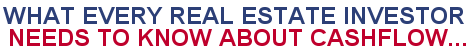Paperback: 261 pages Pub: McGraw-Hill ISBN: 0071422579 Available at:Amazon
Four Annuity Functions (Mortgage Calculations)
 N %i Pmt PV Calculate Payment Calculate Present Value Calculate Number of Periods Calculate Periodic Rate Automatic Recalculation  Four Annuity Functions (Mortgage Calculations) The calculators above will allow you to compute the unknown mortgage variable -- Payment, Present Value, Number of Periods or Periodic Rate -- when you know the other three. N = Number of Periods of the Loan %i = Periodic Interest Rate Pmt = Payment Amount PV = Present Value